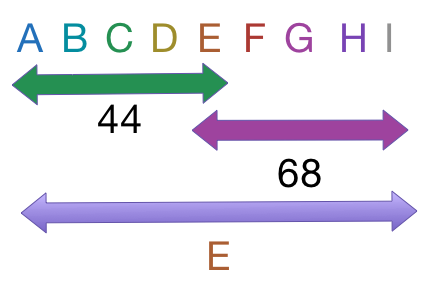# Arpit's awesome averages

Algebra Level 2Nine numbers are written in increasing order. The average of the nine numbers is the middle number, the average of the five largest values is 68, and the average of the five smallest values is 44. What is the sum of the nine numbers?

This problem is posed by Arpit S.

×